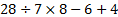## Coding and Decoding For SBI PO : Set – 14

1) If GARDEN is coded as 325764 and WATER as 92165, how can we code the word WARDEN in the same way?

a) 925764

b) 295764

c) 952764

d) 957264

a)

G A R D E N

3 2 5 7 6 4

W A T E R

9 2 1 6 5

W A R D E N

9 2 5 7 6 4

2. In a certain language

A. PIC VIC NIC means winter is cold

B. TO NIC RE means summer is hot

C. RE THO PA means nights are hot

Which of the following is the code for summer?

a) TO

b) NIC

c) PIC

d) VIC

a)

Summer – TO

is     – NIC

Hot  – RE

3. If P denotes multiplied by, T denotes subtracted from;, M denotes added to and B denotes divided by, then 28 B 7 P8 T 6 M 4 = ?

a) -3/2

b) 30

c) 32

d) 34

b)= 30

4) In Certain code, ‘253’ means ‘books are old’; ‘546’ means ‘man is old’ and ‘378’ means ‘buy good books’. What stands for “are” in that code?

a) 2

b) 4

c) 5

d) 6

a)

From above coding we know that

5 means old

3 means book

2 means are

5. In a certain code “MOUSE” is written as “PRUQC”. How is “SHIFT” written in that code ?

a) VJIDR

b) VIKRD

c) RKIVD

d) VKIDR

d)

M+3=P ;O+3=R; U+0=U; S-2=Q; E-2=C like that

S+3=V ;H+3=K;  I+0=I;    F-2=D;T-2=R

6. BHARATHI is written as 2811812089 in a certain code. How would SAMRAT is written as

a) 1911311820

b) 1911318120

c) 1913118120

d) 1911320118

b)

Alphabetical letter with series number

B H A R A T H I

2 8 1 18 1 20 8 9

S A M R A T

19 1 13 18 1 20

7. If ‘+’ means ‘÷’ , ‘÷’ means ‘-’ , ‘-’ means ‘x’ and ‘x’ means ‘+’ then, which of the following equation equal

a) 12×7 – 363 +121=39 /4+6-9

b) 12+7 +363 x121=40/4-6-9

c) 12+7×363 +121=39+4÷6-9

d) 12+7 +363 x121+4+6-9=40

a)

12+7 x 363/121=39-4/6 x 9

33=33

8. IF E=10, PRT=108, then find HWF=?

a) 33

b) 74

c) 88

d) 64

b)

The position of alphabet E is 5, 5*2= 10, then similarly  PRT= 16+18+20=54*2=108, then HWF=8+23+6=37*2=74.

9. in certain code GLITER is written as TERGLI, in that code TAILOR is written as

a) TAIROL

b) IATLOR

c) LORIAT

d) LORTAI

d)

G       L        I        T        E        R

T        E        R        G       L        I

The pattern is 1st three characters are placed at the last part and the last three characters are placed at the first part

T        A        I        L        O       R

L        O       R        T        A        I

10. if E is A, A is R, R is X M is S, T is W, P is D, W is E and D is T. Then what is “WATERED”?

a) XERSWTA

b) ERSXIAW

c) ERWAXAT

d) ETRXWXD